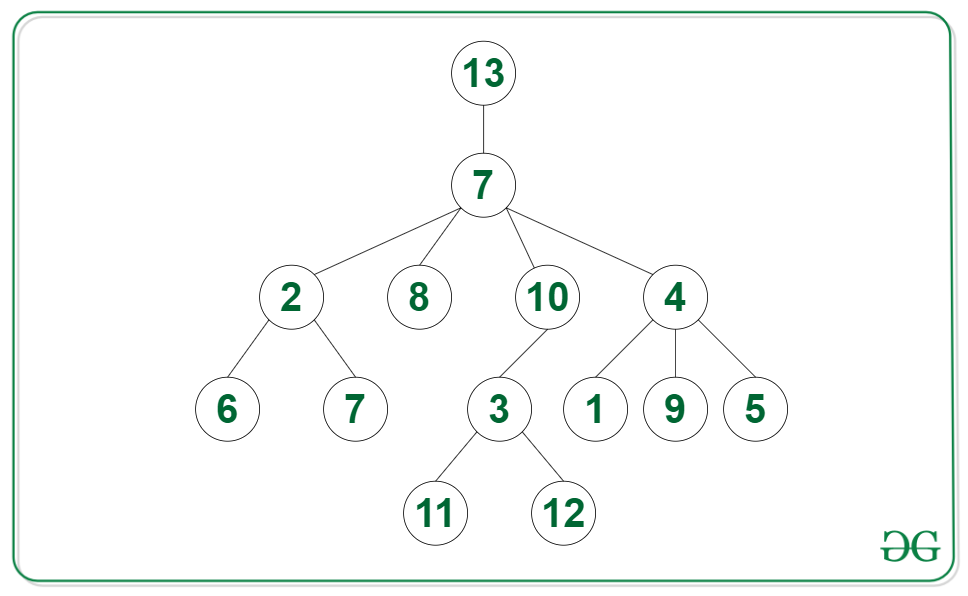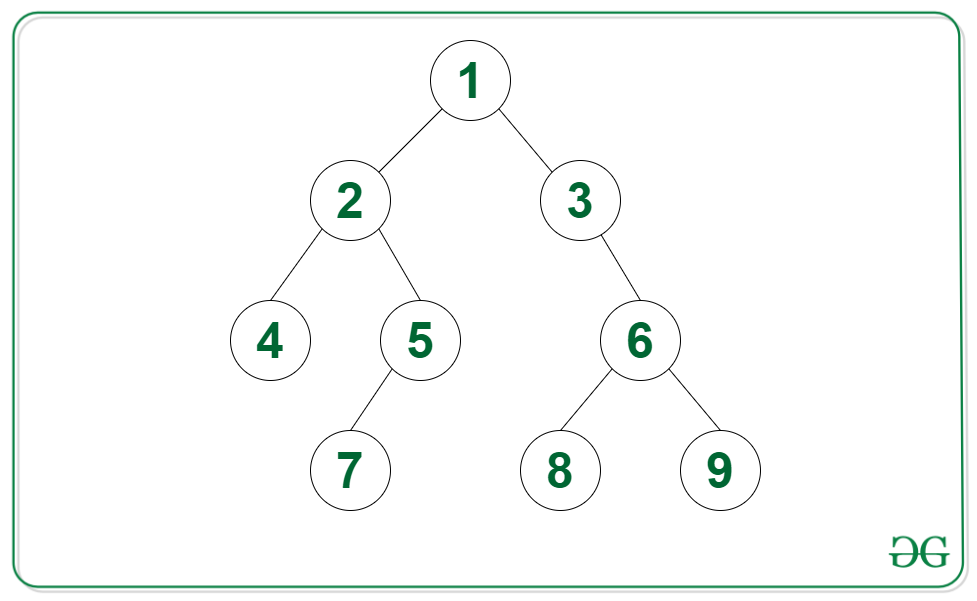Count of nodes in given N-ary tree such that their subtree is a Binary Tree

• Last Updated : 03 Dec, 2021

Given an N-ary tree root, the task is to find the count of nodes such that their subtree is a binary tree.

Example:

Input: Tree in the image belowOutput: 11
Explanation: The nodes such that there subtree is a binary tree are {2, 8, 10, 6, 7, 3, 1, 9, 5, 11, 12}.

Input: Tree in the image belowOutput: 9

Approach: The given problem can be solved by using the post-order traversal. The idea is to use recursion and check if the current node contains at most 2 children and if the children are valid binary trees. Below steps can be followed to solve the problem:

• Apply post-order traversal on the N-ary tree:
• Add the returned values of every child node to calculate the number of binary trees found at that node and store it in sum
• If the root has at most two children which are valid binary trees then the root is also a binary tree, so return a pair of sum + 1 and 1 to indicate a valid binary tree
• If the root has more than two child nodes or any of the children are not valid binary trees, then return the pair of sum and 0 to indicate an invalid binary tree
• Return the value at first index sum, from the pair returned from the post-order traversal.

Below is the implementation of the above approach:

C++

 // C++ code for the above approach #include using namespace std;   class Node { public:     vector children;     int val;       // constructor     Node(int v)     {           val = v;         children = {};     } }; // Post-order traversal to find // depth of all branches of every // node of the tree vector postOrder(Node *root) {       // Initialize a variable sum to     // count number of binary trees     int sum = 0;       // Integer to indicate if the tree     // rooted at current root is a     // valid binary tree     int valid = 1;       // Use recursion on all child nodes     for (Node *child : root->children)     {           // Get the number of binary trees         vector binTrees = postOrder(child);           // If tree rooted at current child         // is not a valid binary tree then         // tree rooted at current root is         // also not a valid binary tree         if (binTrees == 0)             valid = 0;           // If branches are unbalanced         // then store -1 in height         sum += binTrees;     }       // Children are valid binary trees     // and the number of children     // are less than 3     if (valid == 1 && root->children.size() < 3)     {           // Root is also a valid binary tree         sum++;     }       // Children are leaf nodes but number     // of children are greater than 2     else         valid = 0;       // Return the answer     return {sum, valid}; }   // Function to find the number of // binary trees in an N-ary tree int binTreesGeneric(Node *root) {       // Base case     if (root == NULL)         return 0;       // Apply post-order traversal on     // the root and return the answer     return postOrder(root); }   // Driver code int main() {       // Initialize the graph     Node *twenty = new Node(20);     Node *seven = new Node(7);     Node *seven2 = new Node(7);     Node *five = new Node(5);     Node *four = new Node(4);     Node *nine = new Node(9);     Node *one = new Node(1);     Node *two = new Node(2);     Node *six = new Node(6);     Node *eight = new Node(8);     Node *ten = new Node(10);     Node *three = new Node(3);     Node *mfour = new Node(11);     Node *zero = new Node(12);     three->children.push_back(mfour);     three->children.push_back(zero);     ten->children.push_back(three);     two->children.push_back(six);     two->children.push_back(seven2);     four->children.push_back(nine);     four->children.push_back(one);     four->children.push_back(five);     seven->children.push_back(ten);     seven->children.push_back(two);     seven->children.push_back(eight);     seven->children.push_back(four);     twenty->children.push_back(seven);       // Call the function     // and print the result     cout << (binTreesGeneric(twenty)); }   // This code is contributed by Potta Lokesh

Python3

 # Python code for the above approach class Node:     # constructor   def __init__(self, v):     self.val = v;     self.children = [];   # Post-order traversal to find # depth of all branches of every # node of the tree def postOrder(root):     # Initialize a variable sum to   # count number of binary trees   sum = 0;     # Integer to indicate if the tree   # rooted at current root is a   # valid binary tree   valid = 1;     # Use recursion on all child nodes   for child in root.children:       # Get the number of binary trees     binTrees = postOrder(child);       # If tree rooted at current child     # is not a valid binary tree then     # tree rooted at current root is     # also not a valid binary tree     if (binTrees == 0):       valid = 0;       # If branches are unbalanced     # then store -1 in height     sum += binTrees;         # Children are valid binary trees   # and the number of children   # are less than 3   if (valid == 1 and len(root.children) < 3):       # Root is also a valid binary tree     sum += 1         # Children are leaf nodes but number   # of children are greater than 2   else:     valid = 0;     # Return the answer   return [ sum, valid ];     # Function to find the number of # binary trees in an N-ary tree def binTreesGeneric(root):     # Base case   if (root == None):     return 0;     # Apply post-order traversal on   # the root and return the answer   return postOrder(root);     # Driver code   # Initialize the graph twenty = Node(20); seven = Node(7); seven2 = Node(7); five = Node(5); four = Node(4); nine = Node(9); one = Node(1); two = Node(2); six = Node(6); eight = Node(8); ten = Node(10); three = Node(3); mfour = Node(11); zero = Node(12); three.children.append(mfour); three.children.append(zero); ten.children.append(three); two.children.append(six); two.children.append(seven2); four.children.append(nine); four.children.append(one); four.children.append(five); seven.children.append(ten); seven.children.append(two); seven.children.append(eight); seven.children.append(four); twenty.children.append(seven);   # Call the function # and print the result print((binTreesGeneric(twenty)));   # This code is contributed by gfgking

Javascript



Output

11

Time Complexity: O(N)
Auxiliary Space: O(N)

My Personal Notes arrow_drop_up
Recommended Articles
Page :